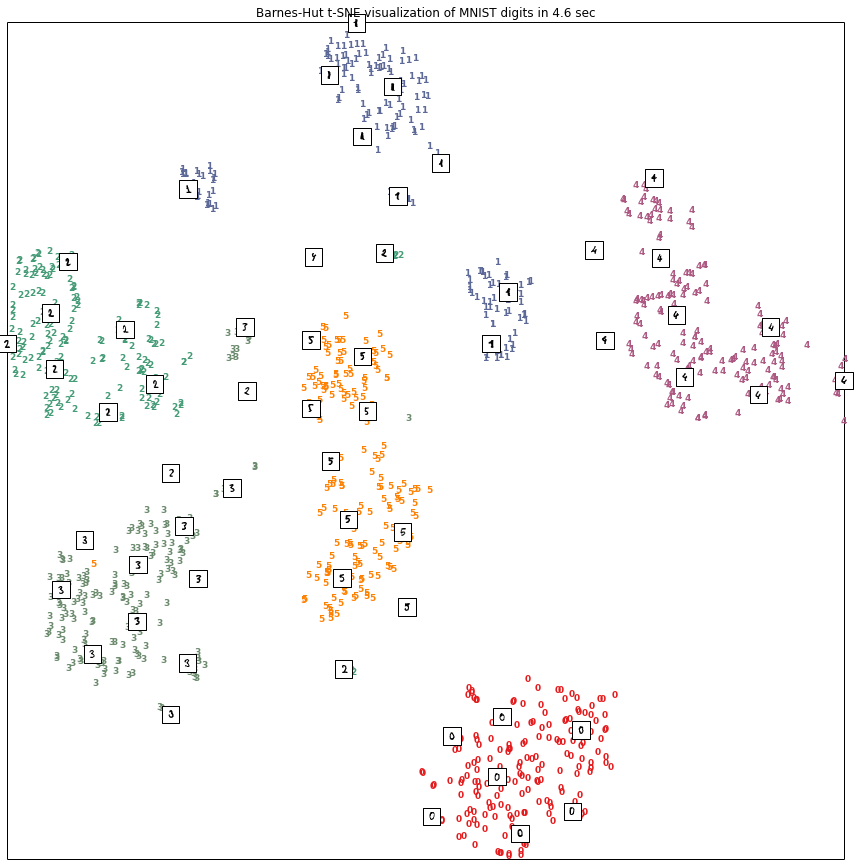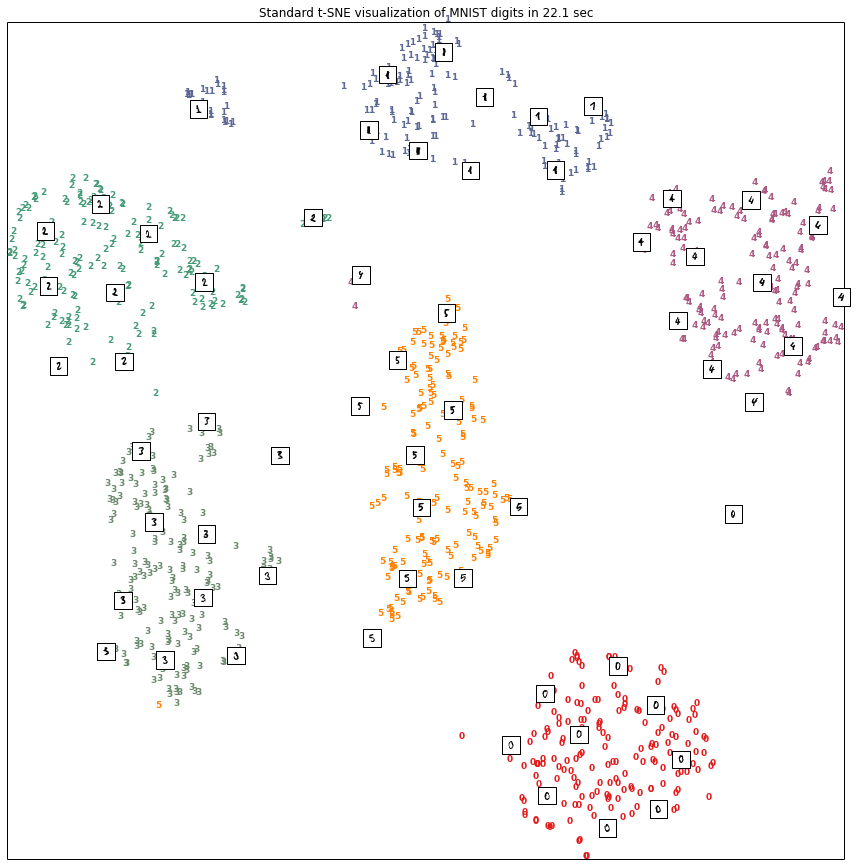## Summary¶

This notebook presents the Barnes-Hut implementation of t-SNE. t-SNE is used to visualize high-dimensional data in a low dimensional space that attempts preserve the pairwise high-dimensional similarities in a low-dimensional embedding. The Barnes-Hut algorithm, which is used by astrophysicists to perform N-body simulations, allows the calculation of the t-SNE embedding in $O(N log N)$ time instead of $O(N^{2})$. This effectively allows us to learn embeddings of data sets with millions of elements instead of tens of thousands.

## Install¶

To try out out the BH version of t-SNE, do the following:

Checkout out scikit-learn

git clone https://github.com/cemoody/scikit-learn.git



Change to my branch

git checkout cemoody/bhtsne



Rebuild the sources, especially the new Cython file sklearn/manifold/bhtsne.pyx

python setup.py build_ext -i



curl https://gist.githubusercontent.com/cemoody/01135ef2f26837548360/raw/57288dd4ebd97a1d6447b14c78b8fb743147b91e/Barnes-Hut%20t-SNE%20Demo.ipynb > bhtsne_demo.ipynb



Start the iPython Notebook, and then open the notebook

ipython notebook



Alternatively, you can download the script version of this and run it:

curl https://gist.githubusercontent.com/cemoody/64ec3934e5b540e8f6e8/raw/f66809381d1baf005a2e2cd6c733e027fbf2e5eb/demo_bhtsne.py > demo_bhtsne.py

## Demo of BH t-SNE¶

In :
%matplotlib inline

In :
from sklearn.manifold import bhtsne
import sys
import numpy as np
import matplotlib.pyplot as plt
from time import time
from matplotlib import offsetbox
from sklearn import (manifold, datasets, decomposition, ensemble, lda, random_projection)
from sklearn.datasets import fetch_mldata

In :
digits = datasets.load_digits(n_class=6)
X = digits.data
y = digits.target
n_samples, n_features = X.shape
n_neighbors = 30

In :
def plot_embedding(X, title=None):
x_min, x_max = np.min(X, 0), np.max(X, 0)
X = (X - x_min) / (x_max - x_min)

plt.figure(figsize=(15,15))
ax = plt.subplot(111)
for i in range(X.shape):
plt.text(X[i, 0], X[i, 1], str(digits.target[i]),
color=plt.cm.Set1(y[i] / 10.),
fontdict={'weight': 'bold', 'size': 9})

if hasattr(offsetbox, 'AnnotationBbox'):
# only print thumbnails with matplotlib > 1.0
shown_images = np.array([[1., 1.]])  # just something big
for i in range(digits.data.shape):
dist = np.sum((X[i] - shown_images) ** 2, 1)
if np.min(dist) < 4e-3:
# don't show points that are too close
continue
shown_images = np.r_[shown_images, [X[i]]]
imagebox = offsetbox.AnnotationBbox(
offsetbox.OffsetImage(digits.images[i], cmap=plt.cm.gray_r),
X[i])
plt.xticks([]), plt.yticks([])
if title is not None:
plt.title(title)

In :
tsne = manifold.TSNE(n_components=2, init='pca', random_state=1, method='barnes_hut', n_iter=1000, verbose=20)
t0 = time()
Xtbh = tsne.fit_transform(X)
t1 = time()
dtbh = t1 - t0

[t-SNE] Computing pairwise distances...
[t-SNE] Computing nearest neighbors...
[t-SNE] Computed conditional probabilities for sample 1000 / 1083
[t-SNE] Computed conditional probabilities for sample 1083 / 1083
[t-SNE] Mean sigma: 11.722976
[t-SNE] Iteration 25: error = 1.0299997, gradient norm = 0.0027466
[t-SNE] Iteration 50: error = 0.9620813, gradient norm = 0.0023000
[t-SNE] Iteration 75: error = 0.8291281, gradient norm = 0.0020988
[t-SNE] Iteration 100: error = 0.7979451, gradient norm = 0.0015836
[t-SNE] Error after 100 iterations with early exaggeration: 0.797945
[t-SNE] Iteration 125: error = 0.7681983, gradient norm = 0.0017382
[t-SNE] Iteration 150: error = 0.7595036, gradient norm = 0.0027530
[t-SNE] Iteration 175: error = 0.7584765, gradient norm = 0.0019935
[t-SNE] Iteration 200: error = 0.7530495, gradient norm = 0.0021226
[t-SNE] Iteration 225: error = 0.7549619, gradient norm = 0.0025411
[t-SNE] Iteration 250: error = 0.7611942, gradient norm = 0.0034922
[t-SNE] Iteration 250: did not make any progress during the last 30 episodes. Finished.
[t-SNE] Error after 250 iterations: 0.761194

In :
plot_embedding(Xtbh, title="Barnes-Hut t-SNE visualization of MNIST digits in %1.1f sec" % dtbh)## Comparison with standard t-SNE¶

In :
tsne = manifold.TSNE(n_components=2, init='pca', random_state=1, method='standard', n_iter=1000, verbose=20)
t0 = time()
Xts = tsne.fit_transform(X)
t1 = time()
dts = t1 - t0

[t-SNE] Computing pairwise distances...
[t-SNE] Computed conditional probabilities for sample 1000 / 1083
[t-SNE] Computed conditional probabilities for sample 1083 / 1083
[t-SNE] Mean sigma: 11.484561
[t-SNE] Iteration 25: error = 13.6114097, gradient norm = 0.0808075
[t-SNE] Iteration 50: error = 13.9680418, gradient norm = 0.0747129
[t-SNE] Iteration 75: error = 13.7644859, gradient norm = 0.0766499
[t-SNE] Iteration 100: error = 14.1081392, gradient norm = 0.0687183
[t-SNE] Error after 100 iterations with early exaggeration: 14.108139
[t-SNE] Iteration 125: error = 0.6980993, gradient norm = 0.0041228
[t-SNE] Iteration 150: error = 0.6599139, gradient norm = 0.0023878
[t-SNE] Iteration 175: error = 0.6607853, gradient norm = 0.0025294
[t-SNE] Iteration 200: error = 0.6592752, gradient norm = 0.0028095
[t-SNE] Iteration 225: error = 0.6612042, gradient norm = 0.0029860
[t-SNE] Iteration 250: error = 0.6650373, gradient norm = 0.0040230
[t-SNE] Iteration 275: error = 0.6749852, gradient norm = 0.0036559
[t-SNE] Iteration 275: did not make any progress during the last 50 episodes. Finished.
[t-SNE] Error after 275 iterations: 0.674985

In :
plot_embedding(Xts, title="Standard t-SNE visualization of MNIST digits in %1.1f sec" % dts)